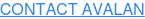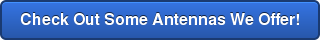By: John Fredrickson on July 28th, 2016

# Understanding the Decibel

When working with wireless, people come across several somewhat confusing concepts. To people who work with wireless day in and day out, this may seem commonplace. Though, to those that are not immersed in the wireless industry, these concepts can seem foreign and complicated. One of these is the decibel (abbreviated dB).

The decibel is not unique to wireless, and is in fact simply a unit of measurement, just like a gram, or a kilometer. Unlike the gram or the kilometer however, a decibel does not measure a physical quantity like mass or distance. The decibel is a measurement of the ratio between two physical quantities, usually power or intensity. Also unlike the gram or the kilometer, the decibel is a logarithmic unit which means that value of the decibel goes up non-linearly. This has the advantage of being able to conveniently express very large or very small numbers. It also allows for the multiplication of ratios (of which the decibel is measuring) by simple addition and subtraction.

Logarithms

I mentioned before that the decibel is a logarithmic unit. What does that mean? To understand decibels you need a basic understanding of logarithms. In mathematics, the logarithm is the inverse operation to exponentiation, where exponentiation is raising a number to a power (giving it an exponent). So for example:

if  1000= 103 then log10(1000) = 3

We can take this a step further in the addition and subtraction of logarithms and say that, for example:

If  103+103= 106, because (10+10+10)+ (10+10+10)= 106  then log10(1000)+log10(1000)= log10(1000×1000)= 6

and

log10(1,000,000)- log10(1000)= log10(1,000,0001000)= log10(1000) = 3

Now, why is all that important? Well, a decibel is a logarithmic unit that expresses ratios, so by adding and subtracting decibels, we are multiplying and dividing ratios.

What is a Decibel?

The decibel was named after Alexander Graham Bell and is commonly used to provide a relative measure of sound intensity. Because of the prefix “deci”, 1 decibel = .1 Bels. But people rarely use the Bel, because 1 decibel is the just noticeable difference (JND) in sound intensity for the normal human ear. Because the decibel is measuring a ratio, to standardize the decibel you must be comparing a value or intensity to a standardized value or intensity. For example, if I0 represents the threshold of hearing (the sound intensity required to be heard by the human ear), and I is the intensity compared to the threshold of hearing, then:

I(dB)= 10log10[II0]

Using this formula you would find the intensity of the sound in decibels with respect to the threshold of hearing.

Decibels in Wireless: dBm, and dBi

So how does the decibel come into play when it comes to wireless? In the world of wireless, rather than intensity we use the decibel to measure a ratio of power. But just like sound, in order to standardize the decibel, the power you are measuring needs to be in relation to a standardized value of power. That is why there are multiple uses of  the decibel in wireless: dBm, dBi, and dBd all have a different value of power that is being compared to.

The first decibel, dBm, is often used to describe transmitters is power relative to 1 milliwatt. Therefore, a transmitter with a power level of 0 dBm corresponds to a power of 1 milliwat, because the ratio is a 1:1 ratio. But to find the power of a 1,000,000mW transmitter, you would use the following formula:

Power in dBm= 10log10[Power in mW1mW]

The result would be that a 1,000,000mW transmitter could be represented as 60dBm. The fact that large numbers can easily be translated into small and manageable numbers is one reason the dBm is used in wireless. Similar to dBm, dBW is also used (with reference to 1 Watt (W)) though not commonly. The dBW is used mainly to describe very large amounts of power.

The dBi is used to describe the forward gain of an antenna. The “i” in dBi stands for isotropic, because it comparing the forward gain of an antenna with a hypothetical isotropic antenna, which uniformly distributes energy in all directions. This antenna is the hypothetical perfect omnidirectional radiator, a point source that does not exist in nature. Knowing the dBi of an antenna is important in wireless, because the power put into the antenna by the transmitter (in dBm) plus the antenna gain (in dBi) is equal to the Effective Isotropic Radiated Power (EIRP) of the radio unit (transmitter plus antenna).

The great thing about the decibel in both of these cases, is that because it is simply a ratio of values, these ratio values can be added and subtracted. So the gain of the antenna can be added to the power of the transmitter, and the loss due to the inefficiencies of cables can be subtracted from that, and so on, to find the link budget. A link budget is an accounting of all the gains and losses between a transmitter and a receiver, and would be much more complicated without the decibel.